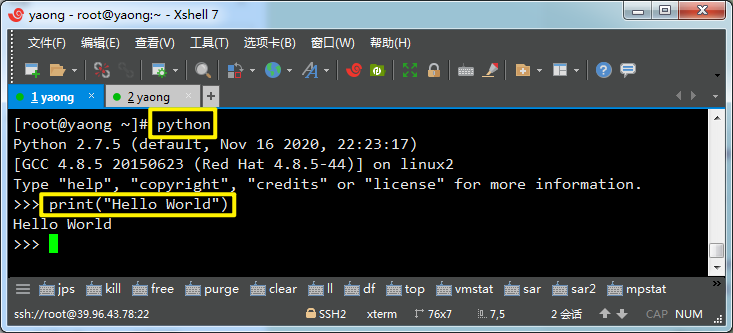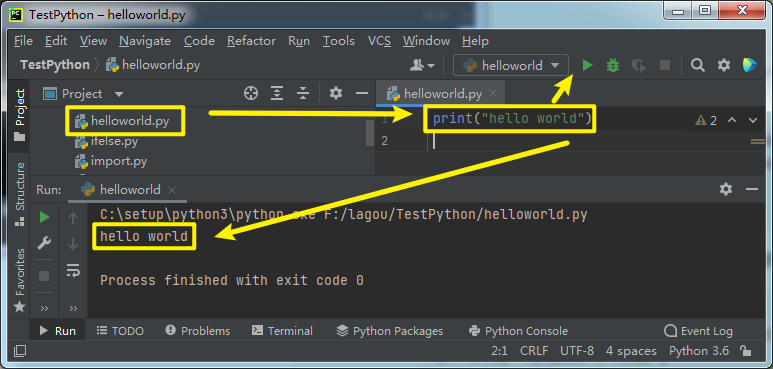# Python入门基础+小套路

2021-08-27 21:35:29  阅读：41  来源： 互联网

## 从开发环境来说主要有两种：

### 1. 命令行交互模式：### 2. 集成开发环境(PyCharm)：## 接入正题

### 变量声明篇

``````x, y = 123,456
print(x, y)
``````

123 456

``````#快捷交换
x, y = y, x
print(x, y)
``````

456 123

### 输出篇

``````print("Hello World")
``````

Hello World

``````print("Hello" , "World")
``````

Hello World

``````print("Hello" + "World")
``````

HelloWorld

``````print("My age is", 18)
``````

My age is 18

``````#ERROR
print("My age is " + 18)
``````
``````Traceback (most recent call last):
File "F:/lagou/TestPython/helloworld.py", line 1, in <module>
print("My age is " + 18)
TypeError: must be str, not int
``````

print("My age is " + str(18))

My age is 18

### 数据类型篇

#### 字符串类型

``````sentence = "This's a very long long long \
long long sentence............"
print(sentence)
``````

This's a very long long long long long sentence............

``````paragraph = """This is line 1,
this is line 2,
this is line 3.
The End.
print(paragraph)
"""
``````

This is line 1,
this is line 2,
this is line 3.
The End.
*

#### 空值类型 None

``````temp = None
print(temp)
``````

None

#### 布尔类型转换

##### 对于数值类型，所有的非零值转换为True, 只有零值才转换为False
``````# 以下值都为True
print(  bool(1)         )
print(  bool(-1)        )
print(  bool(255)       )
print(  bool(0.0000001) )
print(  bool(-99.99)    )
# 下面的值为False
print(  bool(0)         )
print(  bool(0.0)       )
``````
##### 对于任何非空的字符串，转换为布尔值都是True
``````# 这是一个空字符串，转换结果为False
print(  bool("")    )
# 转换结果为True
print(  bool("abc") )
# 这是一个只包含一个空格的字符串，转换结果为为True
print(  bool(" ")   )
``````

False
True
True
*

##### 空值转换为布尔值永远都是False
``````print(  bool(None)  )
``````

False

##### 其他类型 --> 字符串
``````str(True)   # 结果是'True'
str(False)  # 结果是'False'
str(None)   # 结果是'None'
str(123)    # 结果是'123'
``````
##### 字符串 --> 数字
``````int(" 100 ")    # 结果为100
int(3.14)   # 结果为3
float(100)  # 结果为100.0
``````
##### 四舍五入round
``````# 保留小数点后三位，由于第四位是5，所以结果是3.142
round(3.1415926, 3)
``````

### 运算符篇

##### 除和整除
``````print(4 / 2)    # 结果是2.0
print(5 / 2)    # 结果是2.5
print(5 // 2)   # 整除 结果是2
``````
##### 字符串使用运算符
``````print("apple " + "apple " + "apple ")
``````

*apple apple apple *

``````print("apple " * 5)
``````

apple apple apple apple apple

##### 赋值运算符
``````x = 2
y = 3
x **= 3
print(x)
``````

8

### 条件判断篇

##### elif相当于java的else if
``````score = 100
if score >= 60:
if score < 70:
print("您的考试成绩为合格")
elif score < 90:
print("您的考试成绩为良好")
else:
print("您的考试成绩为优秀")
else:
print("您的考试成绩不及格")
``````
##### 简洁复合条件
``````age = 22
if 18 < age < 60:
print("你已经不是个孩子啦，该去工作啦")
``````
##### pass是Python的关键字，表示什么也不做
``````result = None
if result:
pass
else:
print("什么收获都没有")
``````

0值、None 和空字符串转换为布尔值后都是False

### 循环篇

##### wihle循环
``````lap = 0
while lap < 10:
lap += 1
print("我跑完了第" + str(lap + 1) + "圈")
``````

*

##### for循环
``````seq = "hello"
for s in seq:
print(s)
``````

h
e
l
l
o
*

``````for i in range(5):
print(i)
``````

0
1
2
3
4
*

##### 循环嵌套
``````# 指定长方形的宽和高
width, height = 10, 5
# 因为是从上往下开始打印，所以先遍历高度
for i in range(height):
for j in range(width):
print("*", end="")
print()
``````
``````**********
**********
**********
**********
**********
``````
``````for i in range(5):
for j in range(i + 1):
print("*", end="")
print()
``````
``````*
*
**
***
****
*****
``````
``````for i in range(1, 10):
for j in range(1, i + 1):
print("%s*%s=%s" % (j, i, i * j), end=" ")
print()
``````
``````1*1=1
1*2=2 2*2=4
1*3=3 2*3=6 3*3=9
1*4=4 2*4=8 3*4=12 4*4=16
1*5=5 2*5=10 3*5=15 4*5=20 5*5=25
1*6=6 2*6=12 3*6=18 4*6=24 5*6=30 6*6=36
1*7=7 2*7=14 3*7=21 4*7=28 5*7=35 6*7=42 7*7=49
1*8=8 2*8=16 3*8=24 4*8=32 5*8=40 6*8=48 7*8=56 8*8=64
1*9=9 2*9=18 3*9=27 4*9=36 5*9=45 6*9=54 7*9=63 8*9=72 9*9=81
``````